## 2.4 Likelihood considerations

In Section 2.3.4 the cross-validated likelihood was used for bandwidth selection. It is natural to ask why we did not go all-in and simply maximized the likelihood over all possible densities to find a maximum likelihood estimator instead of using the ad hoc idea behind kernel density estimation.

The log-likelihood $\ell(f) = \sum_{i=j}^n \log f(x_j)$ is well defined as a function of the density $$f$$ – even when $$f$$ is not restricted to belong to a finite-dimensional parametric model. To investigate if a nonparametric MLE is meaningful we consider how the likelihood behaves for the densities $\overline{f}_h(x) = \frac{1}{nh \sqrt{2 \pi}} \sum_{j=1}^n e^{- \frac{(x - x_j)^2}{2 h^2} }$ for different choices of $$h$$. Note that $$\overline{f}_h$$ is simply the kernel density estimator with the Gaussian kernel and bandwidth $$h$$.

## The densities can easily be implemented using the density implementation
## of the Gaussian density in R
f_h <- function(x, h) mean(dnorm(x, psi, h))
## This function does not work as a vectorized function as it is, but there is
## a convenience function, 'Vectorize', in R that turns the function into a
## function that can actually be applied correctly to a vector.
f_h <- Vectorize(f_h)

Figure 2.5 shows what some of these densities look like compared to the histogram of the $$\psi$$-angle data. Clearly, for large $$h$$ these densities are smooth and slowly oscillating, while as $$h$$ gets smaller the densities become more and more wiggly. As $$h \to 0$$ the densities become increasingly dominated by tall narrow peaks around the individual data points.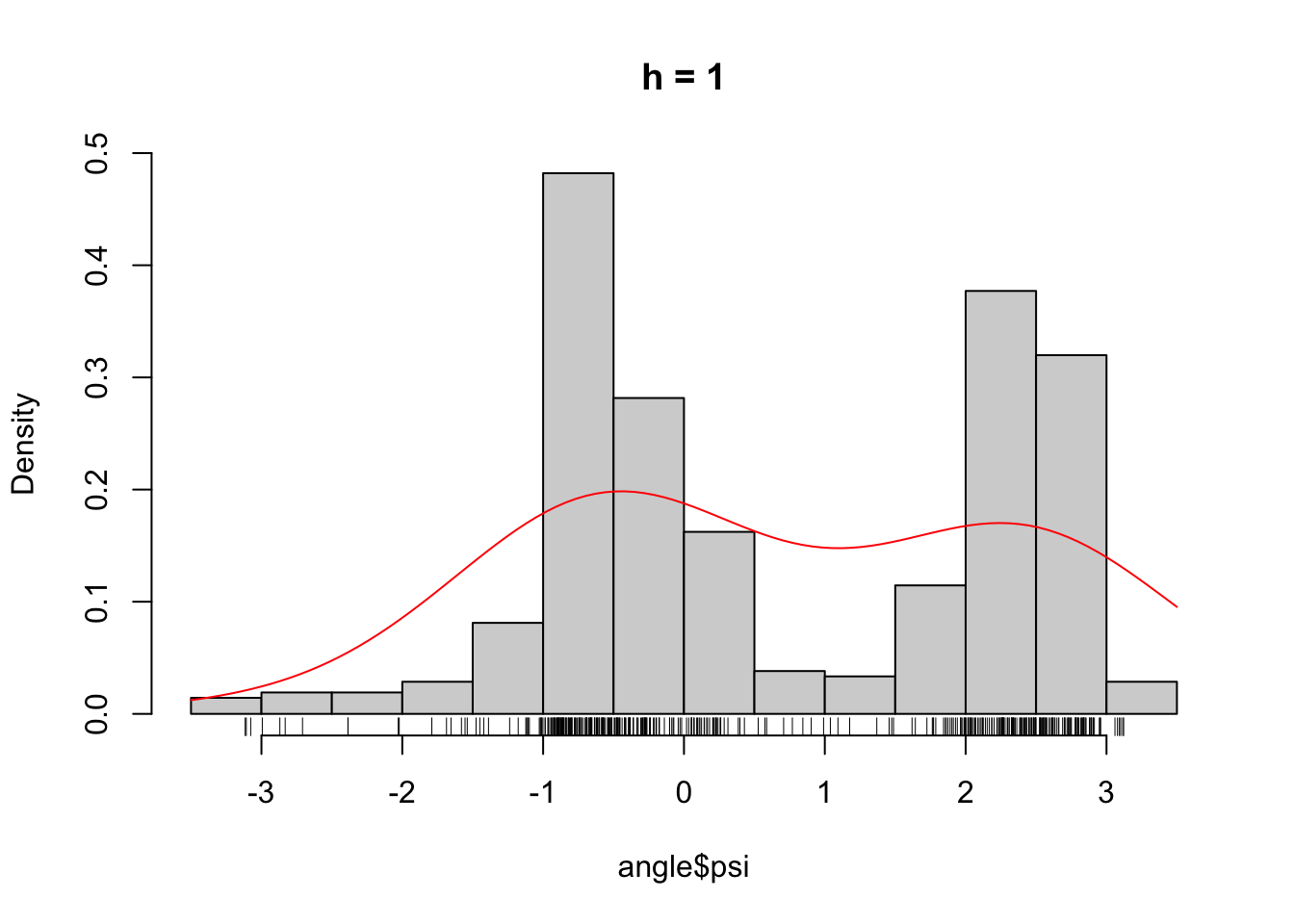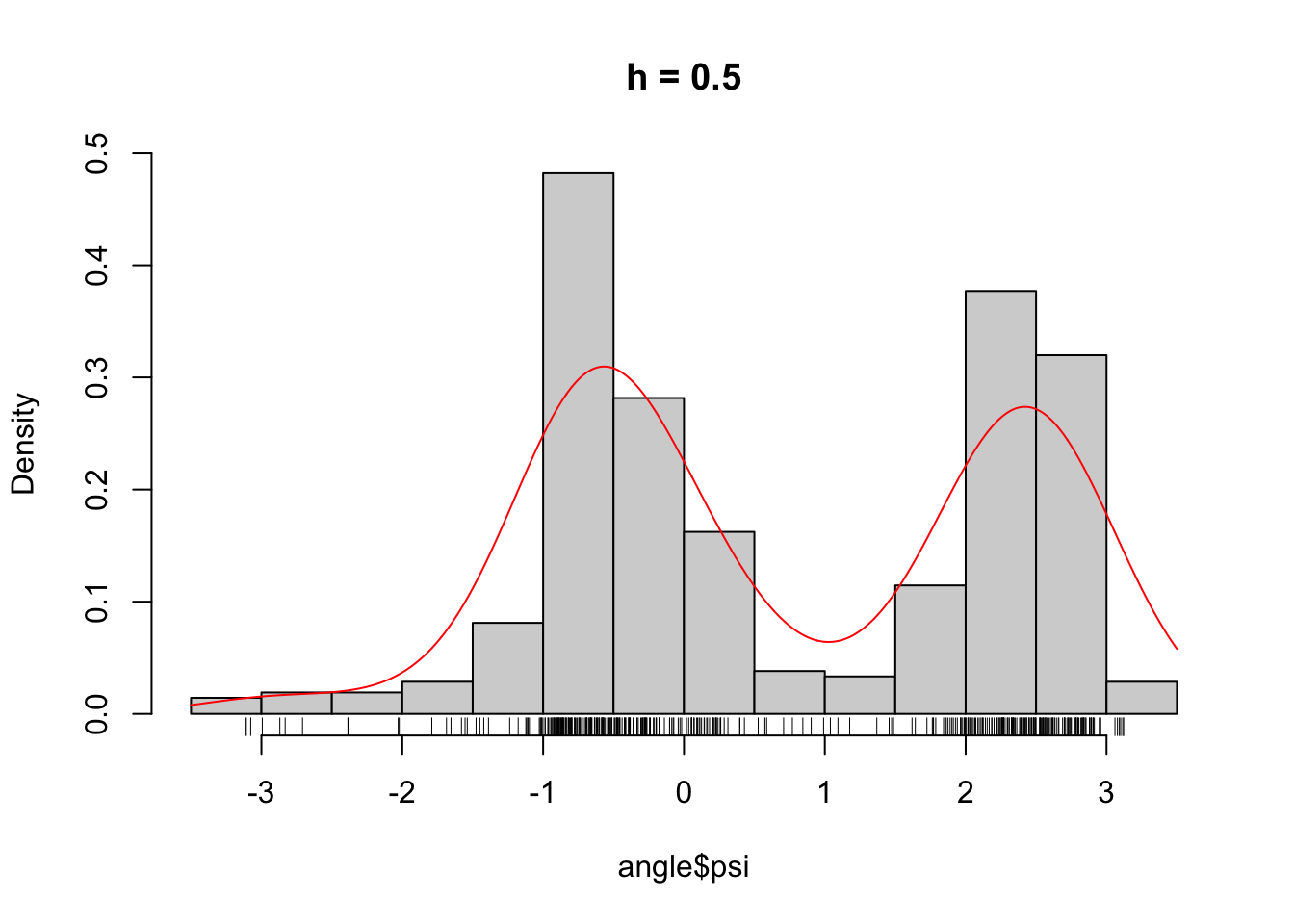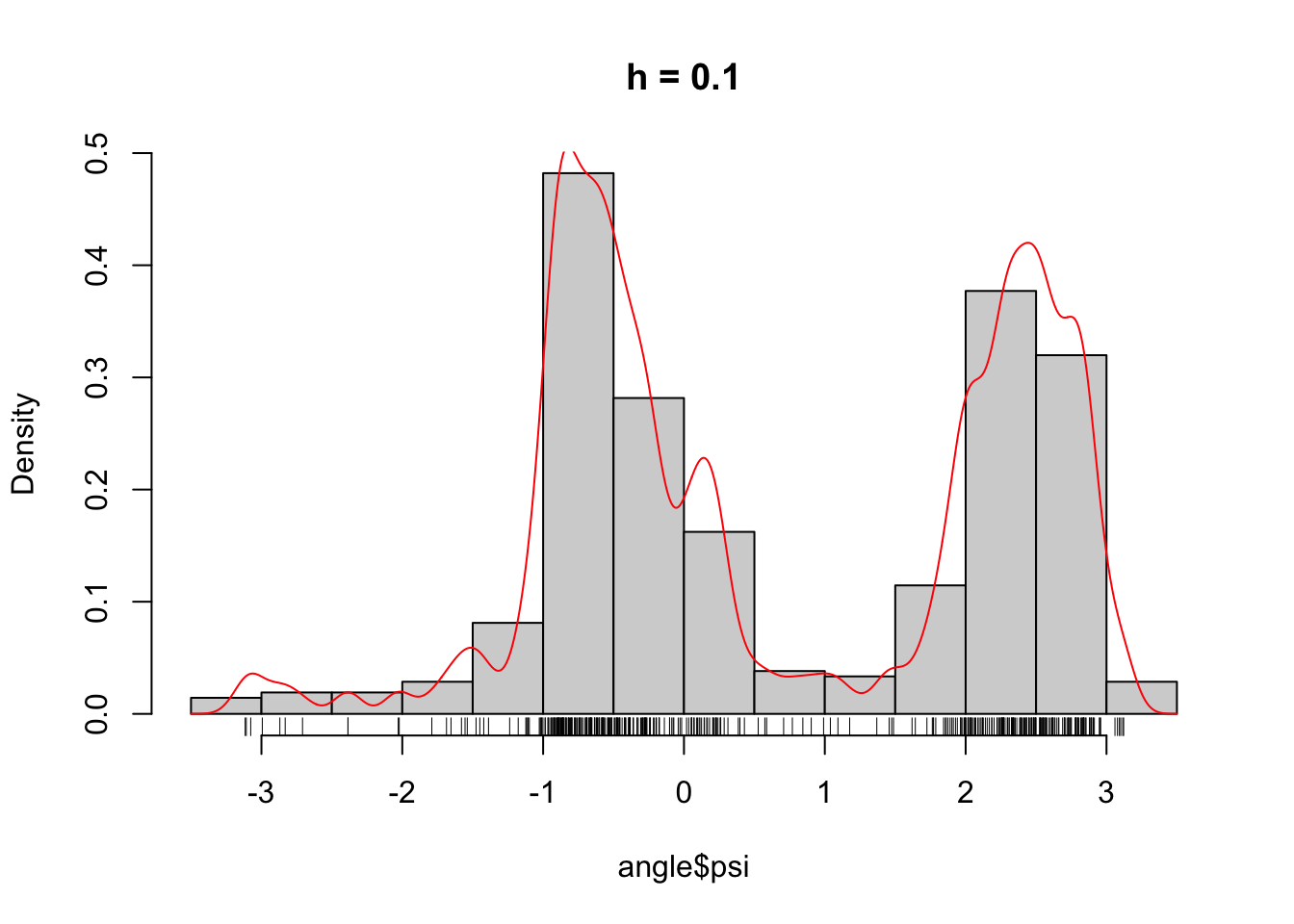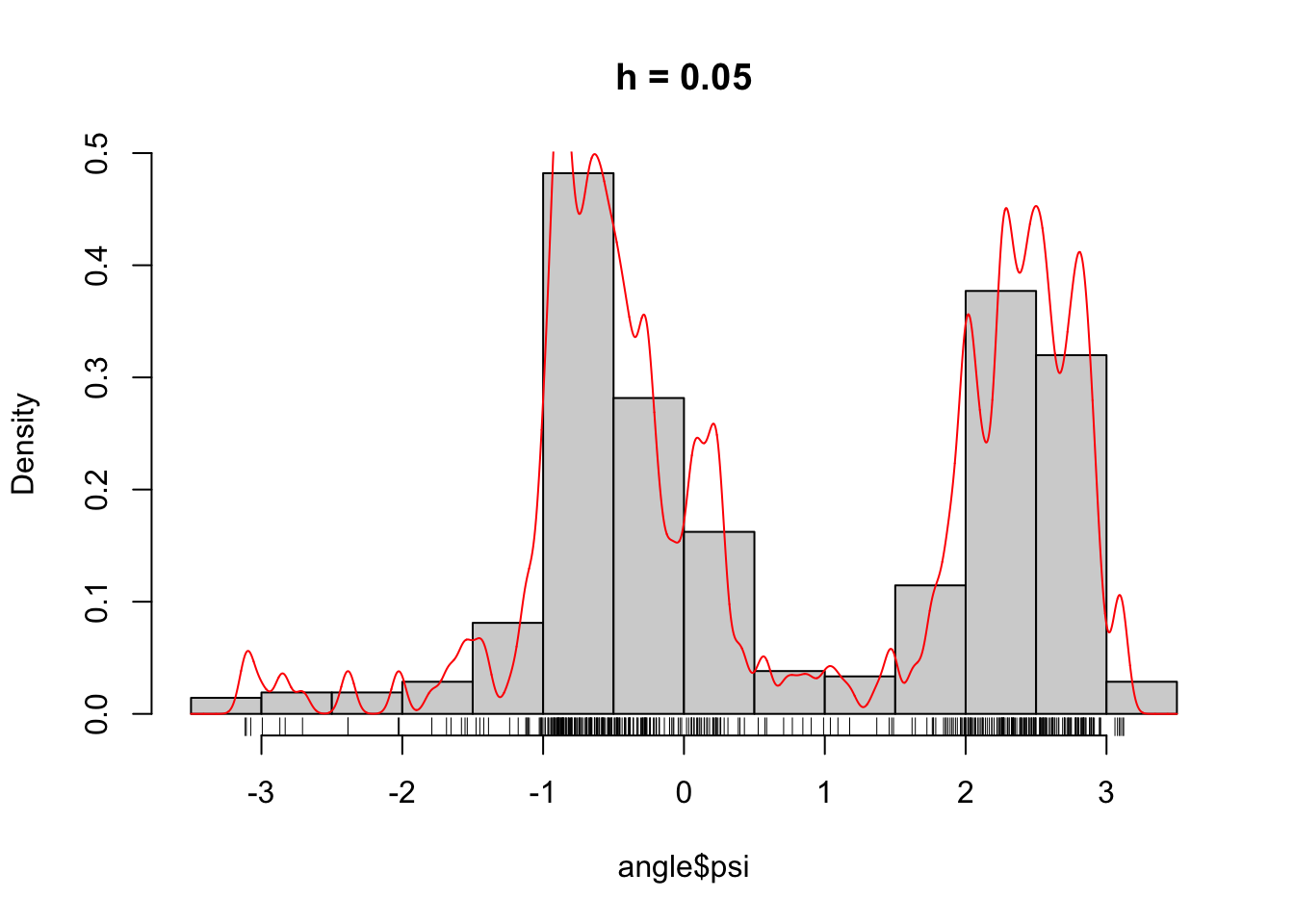Figure 2.5: The densities $$\overline{f}_h$$ for different choices of $$h$$.

The way that these densities adapt to the data points is reflected in the log-likelihood as well.

# To plot the log-likelihood we need to evaluate it in a grid of h-values.
hseq <- seq(1, 0.001, -0.001)
# For the following computation of the log-likelihood it is necessary
# that f_h is vectorized. There are other ways to implement this computation
# in R, and some are more efficient, but the computation of the log-likelihood
# for each h scales as O(n^2) with n the number of data points.
ll <- sapply(hseq, function(h) sum(log(f_h(psi, h))))
qplot(hseq, ll, geom = "line") + xlab("h") + ylab("Log-likelihood")
qplot(hseq, ll, geom = "line") + scale_x_log10("h") + ylab("")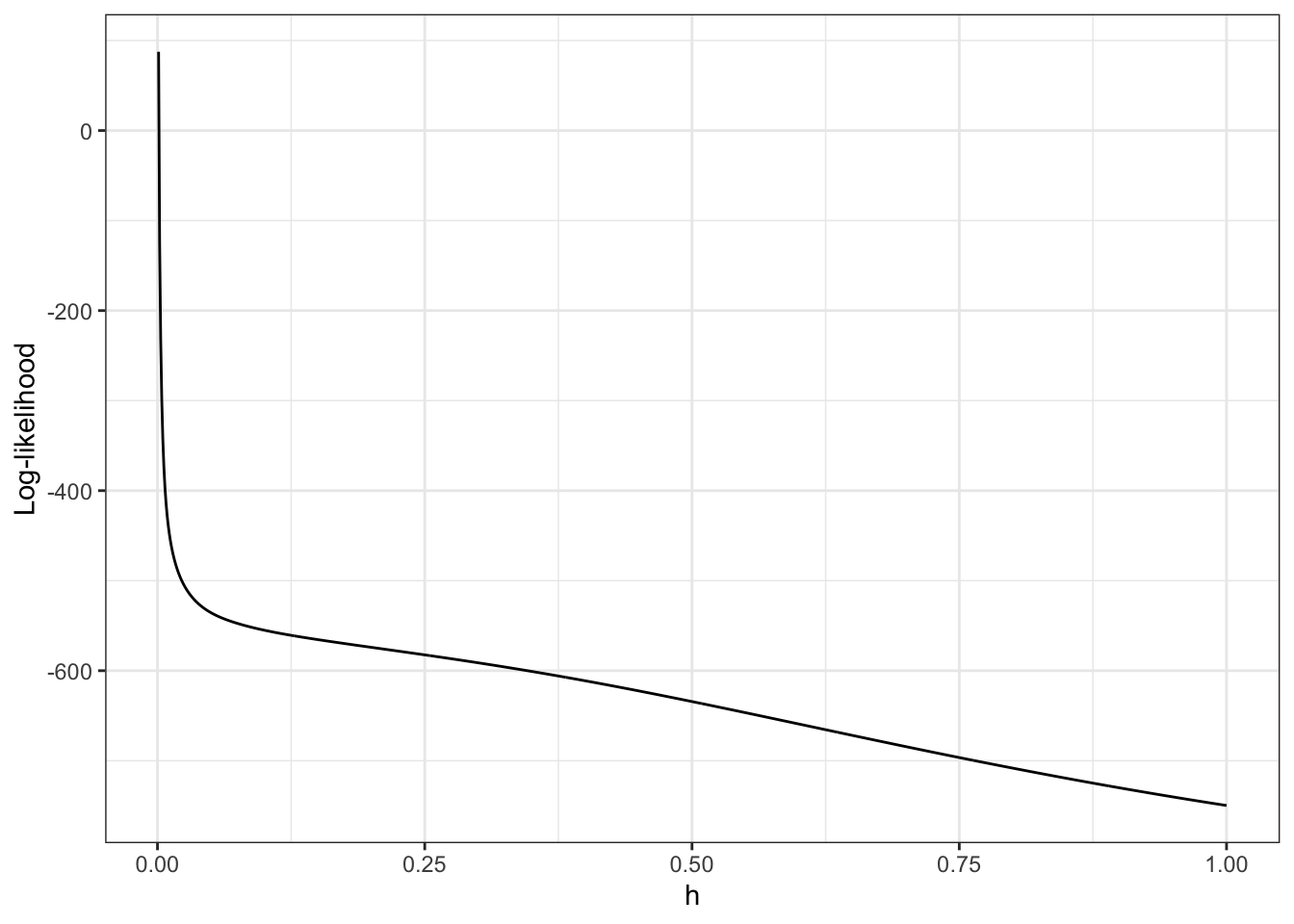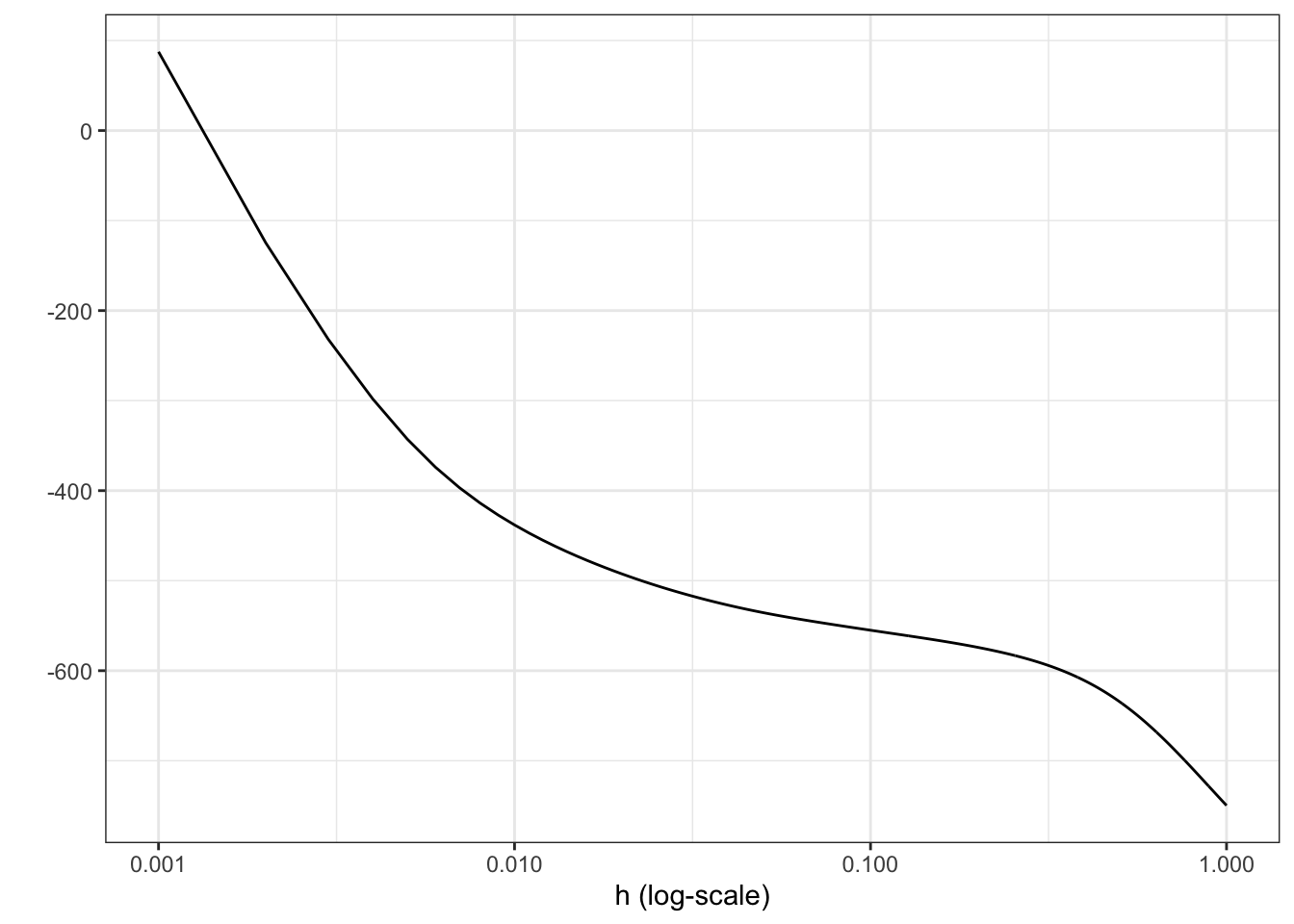Figure 2.6: Log-likelihood, $$\ell(\overline{f}_h)$$, for the densities $$\overline{f}_h$$ as a function of $$h$$. Note the log-scale on the right.

From Figure 2.6 it is clear that the likelihood is decreasing in $$h$$, and it appears that it is unbounded as $$h \to 0$$. This is most clearly seen on the figure when $$h$$ is plotted on the log-scale because then it appears that the log-likelihood approximately behaves as $$-\log(h)$$ for $$h \to 0$$.

We can show that that is, indeed, the case. If $$x_i \neq x_j$$ when $$i \neq j$$

\begin{align*} \ell(\overline{f}_h) & = \sum_{i} \log\left(1 + \sum_{j \neq i} e^{-(x_i - x_j)^2 / (2 h^2)} \right) - n \log(nh\sqrt{2 \pi}) \\ & \sim - n \log(nh\sqrt{2 \pi}) \end{align*}

for $$h \to 0$$. Hence, $$\ell(\overline{f}_h) \to \infty$$ for $$h \to 0$$. This demonstrates that the MLE of the density does not exist in the set of all distributions with densities.

In the sense of weak convergence it actually holds that $\overline{f}_h \cdot m \overset{\mathrm{wk}}{\longrightarrow} \varepsilon_n = \frac{1}{n} \sum_{j=1}^n \delta_{x_j}$ for $$h \to 0$$. The empirical measure $$\varepsilon_n$$ can sensibly be regarded as the nonparametric MLE of the distribution, but the empirical measure does not have a density. We conclude that we cannot directly define a sensible density estimator as a maximum-likelihood estimator.

### 2.4.1 Method of sieves

A sieve is a family of models, $$\Theta_{h}$$, indexed by a real valued parameter, $$h \in \mathbb{R}$$, such that $$\Theta_{h_1} \subseteq \Theta_{h_2}$$ for $$h_1 \leq h_2$$. In this chapter $$\Theta_{h}$$ will denote a set of probability densities. If the increasing family of models is chosen in a sensible way, we may be able to compute the MLE $\hat{f}_h = \text{arg max}_{f \in \Theta_h} \ell(f),$ and we may even be able to choose $$h = h_n$$ as a function of the sample size $$n$$ such that $$\hat{f}_{h_n}$$ becomes a consistent estimator of $$f_0$$.

It is possible to take $\Theta_h = \{ \overline{f}_{h'} \mid h' \leq h \}$ with $$\overline{f}_{h'}$$ as defined above, in which case $$\hat{f}_h = \overline{f}_h$$. We will see in the following section that this is simply a kernel estimator.

A more interesting example is obtained by letting $\Theta_h = \left\{ x \mapsto \frac{1}{h \sqrt{2 \pi}} \int e^{- \frac{(x - z)^2}{2 h^2} } \mathrm{d}\mu(z) \Biggm| \mu \textrm{ a probability measure} \right\},$ which is known as the convolution sieve. We note that $$\overline{f}_h \in \Theta_h$$ by taking $$\mu = \varepsilon_n$$, but generally $$\hat{f}_h$$ will be different from $$\overline{f}_h$$.

We will not pursue the general theory of sieve estimators, but refer to the paper Nonparametric Maximum Likelihood Estimation by the Method of Sieves by Geman and Hwang. In the following section we will work out some more practical details for a particular sieve estimator based on a basis expansion of the log-density.

### 2.4.2 Basis expansions

We suppose in this section that the data points are all contained in the interval $$[a,b]$$ for $$a, b \in \mathbb{R}$$. This is true for the angle data with $$a = -\pi$$ and $$b = \pi$$ no matter the size of the data set, but if $$f_0$$ does not have a bounded support it may be necessary to let $$a$$ and $$b$$ change with the data. However, for any fixed data set we can choose some sufficiently large $$a$$ and $$b$$.

In this section the sieve will be indexed by integers, and for $$h \in \mathbb{N}$$ we suppose that we have chosen continuous functions $$b_1, \ldots, b_h : [a,b] \to \mathbb{R}$$. These will be called basis functions. We then define $\Theta_h = \left\{ x \mapsto \varphi(\boldsymbol{\beta})^{-1} \exp\left(\sum_{k=1}^h \beta_k b_k(x)\right) \Biggm| \boldsymbol{\beta} = (\beta_1, \ldots, \beta_h)^T \in \mathbb{R}^h \right\},$ where $\varphi(\boldsymbol{\beta}) = \int_a^b \exp\left(\sum_{k=1}^h \beta_k b_k(x)\right) \mathrm{d} x.$

The MLE over $$\Theta_h$$ is then given as $\hat{f}_h(x) = \varphi(\hat{\boldsymbol{\beta}})^{-1} \exp\left(\sum_{k=1}^h \hat{\beta}_k b_k(x)\right),$ where

\begin{align*} \hat{\boldsymbol{\beta}} & = \text{arg max}_{\boldsymbol{\beta} \in \mathbb{R}^h} \ \sum_{j=1}^n \sum_{k=1}^h \beta_k b_k(x_j) - \log \varphi(\boldsymbol{\beta}) \\ & = \text{arg max}_{\boldsymbol{\beta} \in \mathbb{R}^h} \ \ \mathbf{1}^T \mathbf{B} \boldsymbol{\beta} - \log \varphi(\boldsymbol{\beta}). \end{align*}

Here $$\mathbf{B}$$ is the $$n \times h$$ matrix with $$B_{jk} = b_k(x_j)$$. Thus for any fixed $$h$$ the model is, in fact, just an ordinary parametric exponential family, though it may not be entirely straightforward how to compute $$\varphi(\boldsymbol{\beta})$$.

Many basis functions are possible. Polynomials may be used, but splines are often preferred. An alternative is a selection of trigonometric functions, for instance
$b_1(x) = \cos(x), b_2(x) = \sin(x), \ldots, b_{2h-1}(x) = \cos(hx), b_{2h}(x) = \sin(hx)$ on the interval $$[-\pi, \pi]$$. In Section 1.2.1 a simple special case was actually treated corresponding to $$h = 2$$, where the normalization constant was identified in terms of a modified Bessel function.

It is worth remembering the following:

“With four parameters I can fit an elephant, and with five I can make him wiggle his trunk.”

— John von Neumann

The Normal-inverse Gaussian distribution has four parameters and the generalized hyperbolic distribution is an extension with five, but von Neumann was probably thinking more in terms of a spline or a polynomial expansion as above with four or five suitably chosen basis functions.

The quote is not a mathematical statement but an empirical observation. With a handful of parameters you already have a quite flexible class of densities that will fit many real data sets well. But remember that a reasonably good fit does not mean that you have found the “true” data generating model. Though data is in some situations not as scarce a resource today as when von Neumann made elephants wiggle their trunks, the quote still suggests that $$h$$ should grow rather slowly with $$n$$ to avoid overfitting.